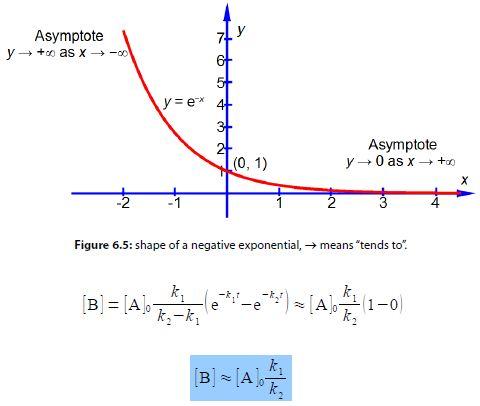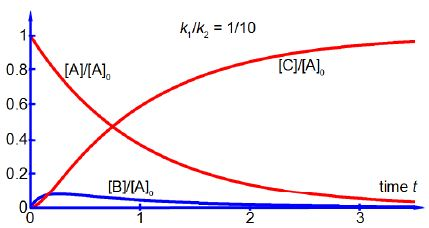Chemistry

# Consecutive Chemical Reactions: the Steady State Approximation

[B] = [A]0 [k1 /(k2 – k1) (e-k1t – e-k2t)

By consideration of each of the terms in the equation show that if k2 is very much larger than k1 i.e. k2 » k1 then [B] and d[B]/dt will be both small and approximately constant.

Now,

When k2 >> k1 then the following approximations may be made. From below Figure we have as k2 is dominantly large then exp (- k2t) ≈ 0 and as k1 is small and positive then exp (- k2t) ≈ exp(0) ≈ 1. Finally (k2 — k1) ≈ k2.As k1/k2 is a small number thus [B] will be small compared to [A]0. Using the same approximations as above with the equation for the rate of forming the intermediate d[B]/dt we have

d[B]/dt = [A]0 [k1 /(k2 – k1) (e-k1t – e-k2t) ≈ [A]0 [k1 /k2]  (e-k1t – e-k2t)

d[B]/dt ≈ – [A]0 (k12 /k2)

As k1/k2 is a small number then k12 /k2 is an even smaller number and thus the rate of change of the [B] concentration is exceedingly small, i.e. [B] is approximately constant. Thus the intermediate concentration [B] is both small and approximately constant under the approximations which follow from where k2 >> k1 and this is called the “Steady State Approximation” in Chemical Reactions kinetics, see below figure.The steady state approximation does not assume the Chemical Reactions intermediate concentration to be constant and therefore its time derivative being zero. The steady state approximation assumes that the variation in the concentration of the intermediate is approximately zero and as the concentration of the intermediate is so low that even a large relative variation in its concentration is small if compared quantitatively against those of the reactant and product.